## 023 Postgres – Ranking and the Timestamp variable

An investigation of ranking / the `timestamp` variable the `time` variable and the `interval` variable.

Hours minutes and seconds
Hours minutes and tenths of seconds
Hours minutes and hundredths of seconds
Hours minutes and thousandths of seconds

So to highlight the examples I will first create a databsae called timeexampledb

`CREATE database timeexampledb;`

Now lets connect to that database

`\c timeexampledb`

Now I create a table called timebucket that will hold examples of the different time formats.

```create table timebucket
(pkid serial primary key,
time1secondonly timestamp(0),
time2tenthsecond timestamp(1),
time3hundredthsecond timestamp(2),
time4timethousandthsecond timestamp(3));
```

Next input some examples and see what we get.

```insert into timebucket values (1, now(),now(),now(),now());
insert into timebucket values (2, now(),now(),now(),now());
insert into timebucket values (3, now(),now(),now(),now());
insert into timebucket values (4, now(),now(),now(),now());
insert into timebucket values (5, now(),now(),now(),now());
insert into timebucket values (6, now(),now(),now(),now());
insert into timebucket values (7, now(),now(),now(),now());
insert into timebucket values (8, now(),now(),now(),now());
insert into timebucket values (9, now(),now(),now(),now());
insert into timebucket values (10, now(),now(),now(),now());
insert into timebucket values (11, now(),now(),now(),now());
insert into timebucket values (12, now(),now(),now(),now());
insert into timebucket values (14, now(),now(),now(),now());
```

and lets see what that looks like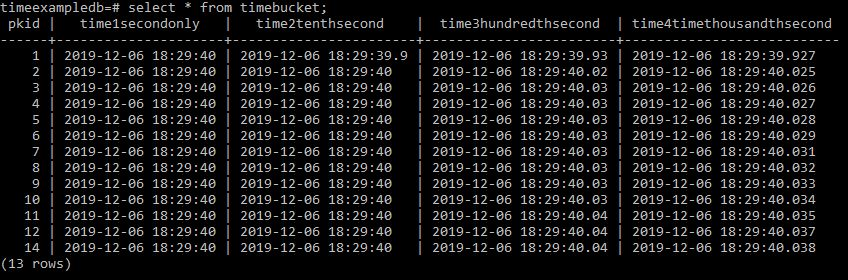Here you can see from the tenth of a second options where you hit right on a second then a digit will disappear.

Now we can do ranking on these to determine position.

```Select pkid,
time1secondonly,
rank() over wn as rank from timebucket
window wn as (order by time1secondonly)
order by time1secondonly;```

This results in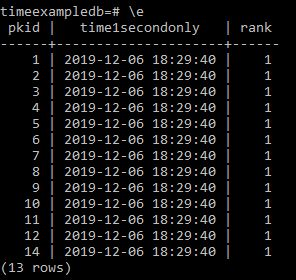So lets change this to rank the next column along.

```Select pkid,
time2tenthsecond,
rank() over wn as rank from timebucket
window wn as (order by time2tenthsecond)
order by time2tenthsecond;```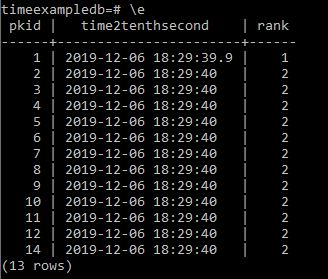Appears to be working but lets try the other columns.

```Select pkid,
time3hundredthsecond,
rank() over wn as rank from timebucket
window wn as (order by time3hundredthsecond)
order by time3hundredthsecond;```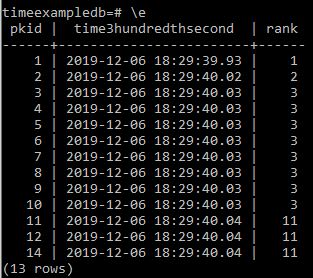Appears correct but for good measure thousandths of a second.

```Select pkid,
time4timethousandthsecond,
rank() over wn as rank from timebucket
window wn as (order by time4timethousandthsecond)
order by time4timethousandthsecond;```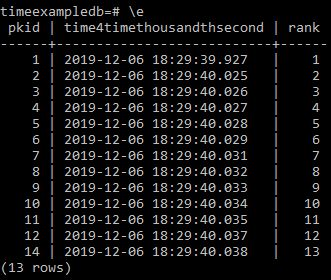And now lets add an interval column

```Alter table timebucket add column timeinterval time(0);
```

But lets add a further time5 column that and update to now time so we can create some intervals

```Alter table timebucket add column time5 timestamp(0);
Update timebucket set time5 = now();
```

Now if we want to get the time between items we can make the following SQL

```Select pkid,
time5,
time1secondonly,
time5-time1secondonly as tinterval
from timebucket;
```

And we get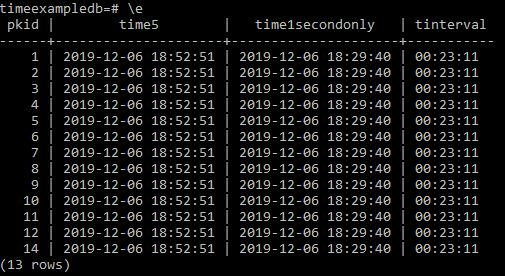Lets try with a different time column

```Select pkid,
time5,
time4timethousandthsecond,
time5- time4timethousandthsecond as tinterval
from timebucket;```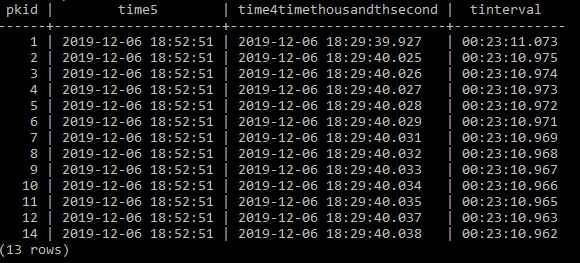So next I reduce pkid record 14 by a day and re run to see what happens.

```Update timebucket set time4timethousandthsecond='2019-12-04' where pkid=14;
```

and run the former select again;

```Select pkid,
time5,
time4timethousandthsecond,
time5- time4timethousandthsecond as tinterval
from timebucket;```

and we see the interval is correctly recording.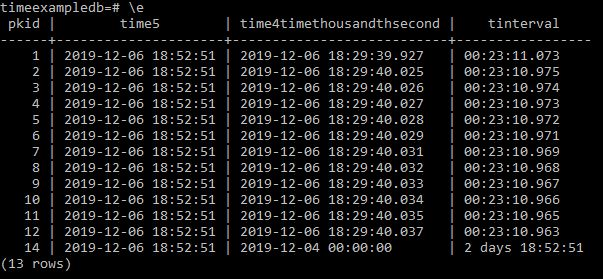Now if we want to rank on tinterval I was unable to do it directly from a query so I went ahead and updated the former timeinterval column as follows

`update timebucket set timeinterval=time5-time4timethousandthsecond;`

and now doing a select on this we get

`select pkid, timeinterval from timebucket;`

What we see is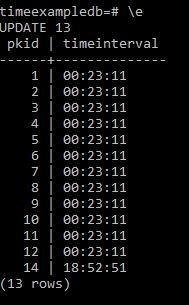But we are not showing the fact that 14 should be 1 day this is because we should have defined `timeinterval` as an `interval` variable rather than a `time(0)` variable.

So we can do this as follows and update appropriately.

```Alter table timebucket add column timeinterval2 interval;
update timebucket set timeinterval2=time5-time4timethousandthsecond;
select pkid, timeinterval2 from timebucket;```

And we get the right resultAnd now lets rank these to check it is sorting them correctly.

```Select pkid,
time4timethousandthsecond,
timeinterval2,
rank() over wn as rank from timebucket
window wn as (order by timeinterval2)
order by rank;```

And we get the correct result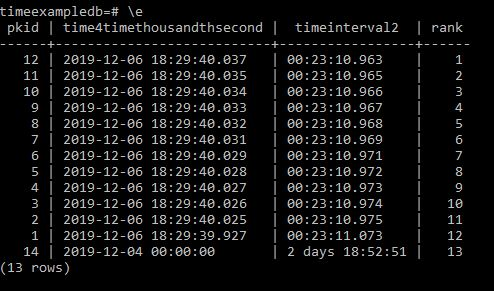## 022 Postgres – Setting up starting variables in psqlrc

So how do we adjust the defaults for the command line prompt in psql Postgres

Go to the command prompt and navigate to the following directory

c:\Users\Mark\AppData\Roaming\Postgresql\

and either find or create a file called

`psqlrc.conf`

This is a simple text file that you can edit or create in notepad.

```--------------------------
--psqlrc set preferences--
-- Author Mark Brooks --
--------------------------

\set QUIET 1

\x auto
\timing
\set COMP_KEYWORD_CASE upper

\pset border 2
\pset pager off
\pset null <NULL>
This allows you for instance to set up which editor will appear when you perform the `\e` command